Next: Plot interpretation Up: Discrete Fourier transform Previous: FT by Z-transform

# SYMMETRIES

Next we examine odd/even symmetries to see how they are affected in Fourier transform. The even part et of a signal bt is defined as(12)
The odd part is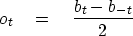(13)
By adding (12) and (13), we see that a function is the sum of its even and odd parts:(14)

Consider a simple, real, even signal such as (b-1, b0, b1) = (1, 0, 1). Its transformis an even function of, since.

Consider the real, odd signal (b-1, b0, b1) = (-1, 0, 1). Its transform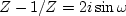is imaginary and odd, since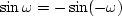.

Likewise, the transform of the imaginary even function (i, 0, i) is the imaginary even function.Finally, the transform of the imaginary odd function (-i, 0, i) is real and odd.

Let r and i refer to real and imaginary, e and o to even and odd, and lower-case and upper-case letters to time and frequency functions. A summary of the symmetries of Fourier transform is shown in Figure 6.

 reRE Figure 5 Odd functions swap real and imaginary. Even functions do not get mixed up with complex numbers.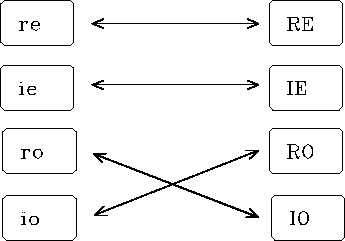More elaborate signals can be made by adding together the three-point functions we have considered. Since sums of even functions are even, and so on, the diagram in Figure 6 applies to all signals. An arbitrary signal is made from these four parts only, i.e., the function has the form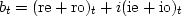.On transformation of bt, each of the four individual parts transforms according to the table.

Most industry standard'' methods of Fourier transform set the zero frequency as the first element in the vector array holding the transformed signal, as implied by equation (3). This is a little inconvenient, as we saw a few pages back. The Nyquist frequency is then the first point past the middle of the even-length array, and the negative frequencies lie beyond. Figure 7 shows an example of an even function as it is customarily stored.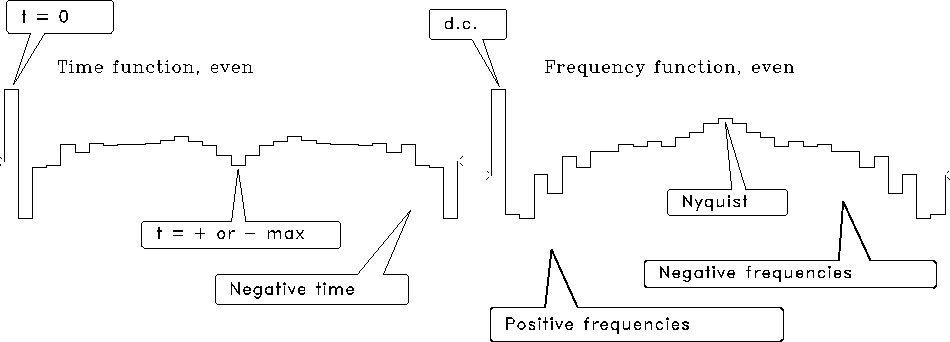even
Figure 6
Even functions as customarily stored by industry standard'' FT programs.Next: Plot interpretation Up: Discrete Fourier transform Previous: FT by Z-transform
Stanford Exploration Project
10/21/1998